# 十四、K 最近邻

## 确定 K 的最佳值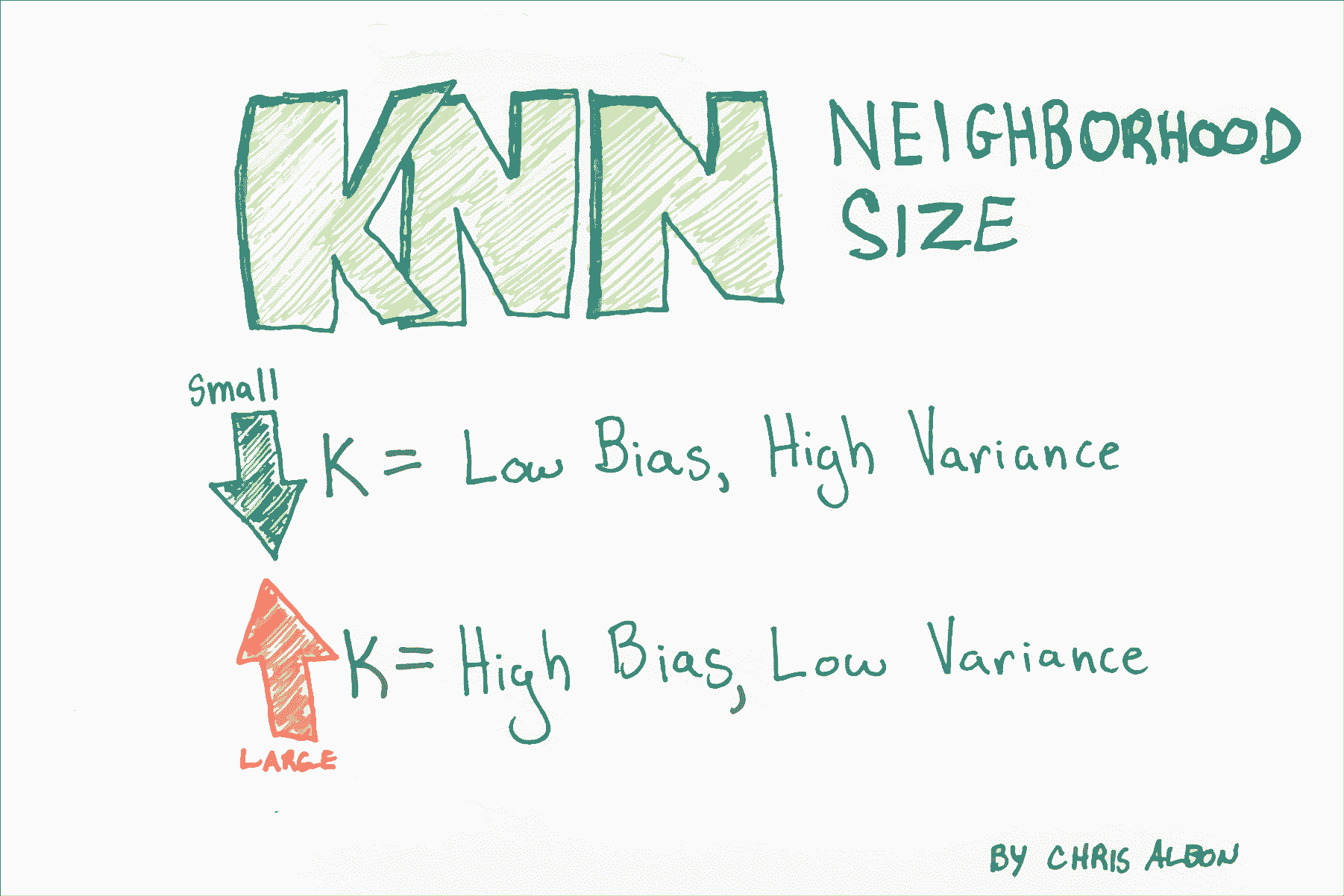``````# 加载库
from sklearn.neighbors import KNeighborsClassifier
from sklearn import datasets
from sklearn.preprocessing import StandardScaler
from sklearn.pipeline import Pipeline, FeatureUnion
from sklearn.model_selection import GridSearchCV

# 加载数据
X = iris.data
y = iris.target

# 创建标准化器
standardizer = StandardScaler()

# 标准化特征
X_std = standardizer.fit_transform(X)

# 拟合 5 个邻居的 KNN 分类器
knn = KNeighborsClassifier(n_neighbors=5, metric='euclidean', n_jobs=-1).fit(X_std, y)

# 创建流水线
pipe = Pipeline([('standardizer', standardizer), ('knn', knn)])

# 创建候选值空间
search_space = [{'knn__n_neighbors': [1, 2, 3, 4, 5, 6, 7, 8, 9, 10]}]

# 创建网格搜索
clf = GridSearchCV(pipe, search_space, cv=5, verbose=0).fit(X_std, y)

# 最佳邻居大小（K）
clf.best_estimator_.get_params()['knn__n_neighbors']

# 6
``````

## KNN 分类

K 最近邻分类器（KNN）是一种简单而强大的分类学习器。

KNN 有三个基本部分

•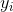: 观测的类别（我们试图在测试数据中预测的东西）。
•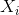: 观察的预测因子/ IV /属性。
•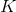: 研究者指定的正数。 K 表示最接近特定观测的观测数，它定义了“邻域”。 例如，`K = 2`意味着每个观测都有一个邻域，包含最接近它的另外两个观测。

``````import pandas as pd
from sklearn import neighbors
import numpy as np
%matplotlib inline
import seaborn
``````

``````training_data = pd.DataFrame()

training_data['test_1'] = [0.3051,0.4949,0.6974,0.3769,0.2231,0.341,0.4436,0.5897,0.6308,0.5]
training_data['test_2'] = [0.5846,0.2654,0.2615,0.4538,0.4615,0.8308,0.4962,0.3269,0.5346,0.6731]
training_data['outcome'] = ['win','win','win','win','win','loss','loss','loss','loss','loss']

``````
test_1 test_2 outcome
0 0.3051 0.5846 win
1 0.4949 0.2654 win
2 0.6974 0.2615 win
3 0.3769 0.4538 win
4 0.2231 0.4615 win

``````seaborn.lmplot('test_1', 'test_2', data=training_data, fit_reg=False,hue="outcome", scatter_kws={"marker": "D","s": 100})

# <seaborn.axisgrid.FacetGrid at 0x11008aeb8>
```````scikit-learn`库需要将数据格式化为`numpy`数组。 这是重新格式化的代码。

``````X = training_data.as_matrix(columns=['test_1', 'test_2'])
y = np.array(training_data['outcome'])
``````

``````clf = neighbors.KNeighborsClassifier(3, weights = 'uniform')
trained_model = clf.fit(X, y)
``````

``````trained_model.score(X, y)

# 0.80000000000000004
``````

``````# 使用 'test_1' 第一个和第二个自变量的值
# 创建一个新观测，为 .4 和 .6
x_test = np.array([[.4,.6]])

# 将学习者应用于新的未分类的观测。
trained_model.predict(x_test)

# array(['loss'], dtype=object)
``````

``````trained_model.predict_proba(x_test)

# array([[ 0.66666667,  0.33333333]])
``````

## 注

• K 的选择对创建的分类器有重大影响。
• K 越大，决策边界越线性（高偏差和低方差）。
• 有多种方法可以测量距离，两种流行的方法是简单的欧几里德距离和余弦相似度。

# 基于半径的 KNN 分类器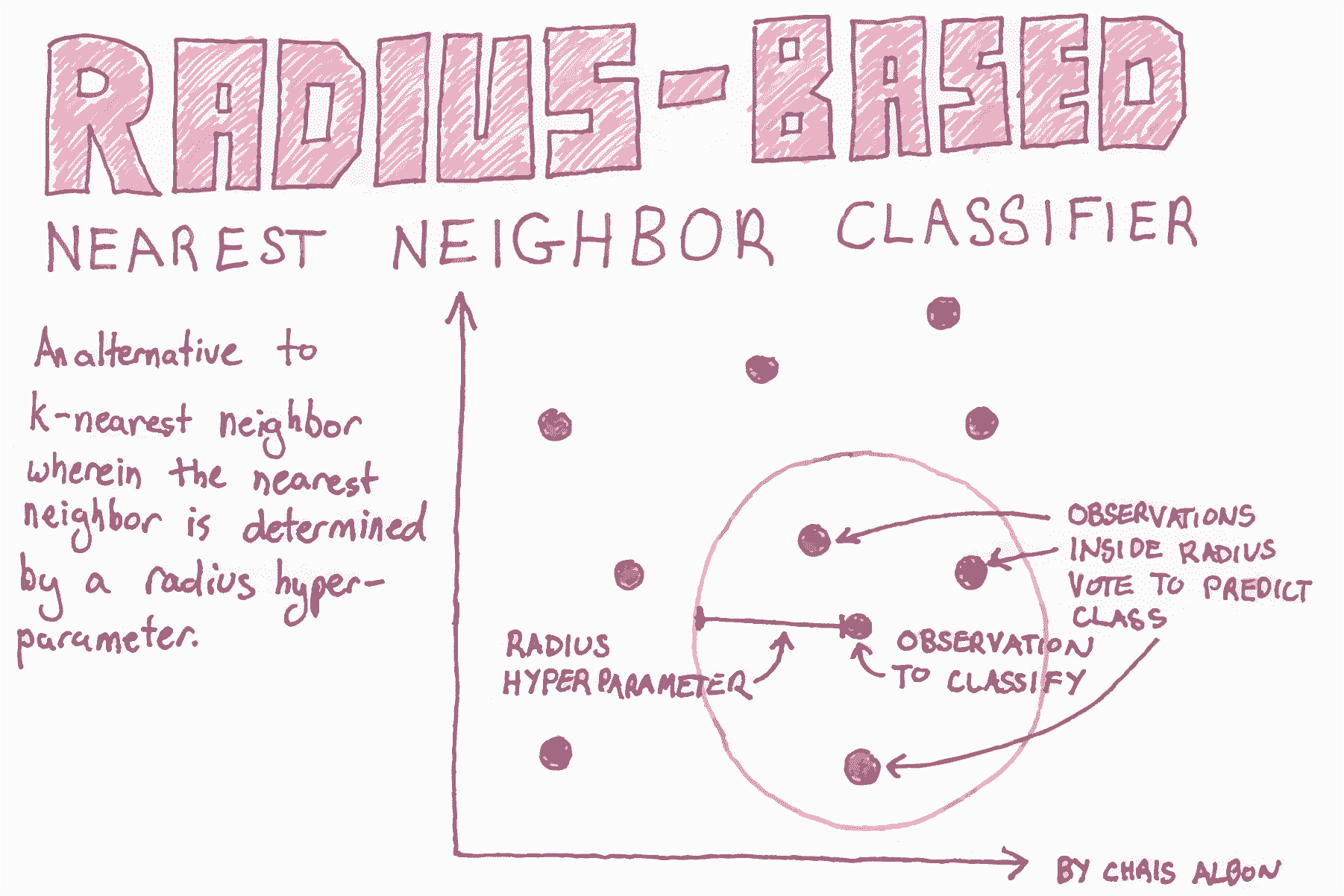``````# 加载库
from sklearn.preprocessing import StandardScaler
from sklearn import datasets

# 加载数据
X = iris.data
y = iris.target

# 创建标准化器
standardizer = StandardScaler()

# 标准化特征
X_std = standardizer.fit_transform(X)
``````

``````# 训练半径邻居分类器